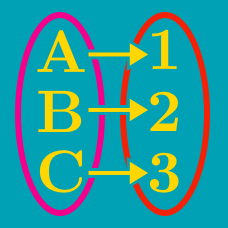Probability

# Bijective Functions

Let $X$ and $Y$ be the sets \begin{aligned} X &= \{ x \mid -12 \leq x \leq 4, x \text{ is an integer} \} \\ Y &= \{ y \mid 4 < y < a, y \text{ is an integer} \} \end{aligned} for some integer $a$. If there exists a bijective function from $X$ to $Y$, what is the value of $a?$

Consider the sets $X=\{a,b,c,d\}, Y=\{7, 13, 19,25\},$ and let $f$ be a bijective function from $X$ to $Y$. If $M$ and $m$ are the maximum and minimum values of $f(a)+f(b)$, respectively, what is $M-m$?

For two sets $X=\{x \mid -1 \leq x \leq 1\}, Y=\{y \mid 2 \leq y \leq 16\},$ a bijective function $f: X \to Y$ is defined as $f(x)=ax+b$, where $a > 0$. What is $ab$?

For set $A=\{-5, 3, 8, 18\}$, how many functions from $A$ to $A$ are not bijective?

For two sets $X=\{x\mid x\geq 11\}, Y=\{y\mid y\geq 121\},$ function $f: X \to Y$ is defined as $f(x)=x^2-2x+a.$ If $f$ is a one-to-one, bijective function, what is the value of the constant $a$?

×